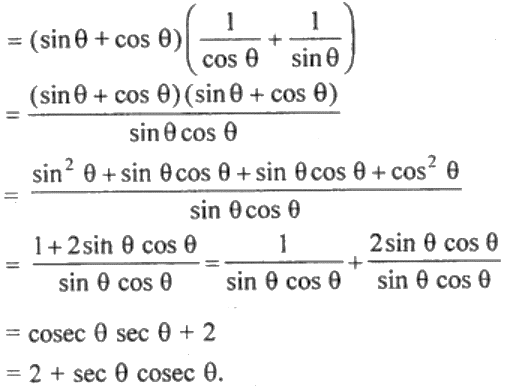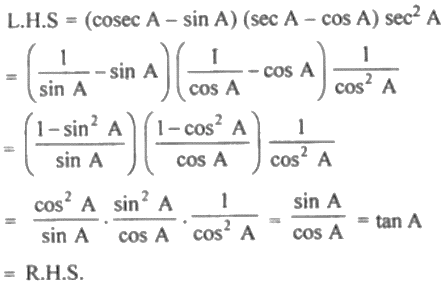Guru

# (i) (sin θ + cos θ) (sec θ + cosec θ) = 2 + sec θ cosec θ (ii) (cosec A – sin A) (sec A – cos A) sec2A = tan A

• 0

This is an important question from the
Book- ML Aggarwal
Board- ICSE
Publication- Avichal
Chapter- Trigonometric Identities
Chapter number-18

We have to prove that equality

(i) (sin θ + cos θ) (sec θ + cosec θ) = 2 + sec θ cosec θ (ii) (cosec A – sin A) (sec A – cos A) sec2A = tan A

Trigonometric Identities, class 10th, chapter 18th, question no 28, ICSE board, ML aggarwal..

Share

1. Solution:

(i) (sin θ + cos θ) (sec θ + cosec θ) = 2 + sec θ cosec θ

L.H.S. = (sin θ + cos θ) (sec θ + cosec θ)= R.H.S.

(ii)• 0